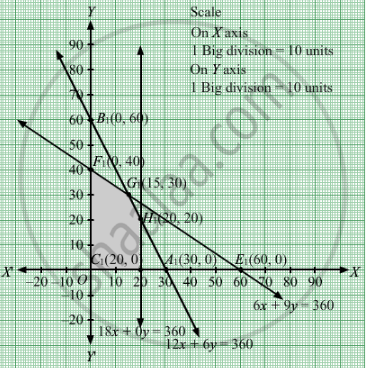# A Manufacturer Makes Two Types a and B of Tea-cups. Three Machines Are Needed for the Manufacture and the Time in Minutes Required for Each Cup on the Machines is Given Below: - Mathematics

Sum

A manufacturer makes two types A and B of tea-cups. Three machines are needed for the manufacture and the time in minutes required for each cup on the machines is given below:

 Machines I II III AB 126 180 69

Each machine is available for a maximum of 6 hours per day. If the profit on each cup A is 75 paise and that on each cup B is 50 paise, show that 15 tea-cups of type A and 30 of type B should be manufactured in a day to get the maximum profit.

#### Solution

Let units of type A and y units of type cups were made.
Quantities cannot be negative.Therefore, $x, y \geq 0$

As we are given,

 Machines I II III AB 126 180 69
Every machine is available for a maximum of 6 hours per day i.e. 360 minutes per day.
Therefore, the constraints are

$12x + 6y \leq 360$
$18x + 0y \leq 360$
$6x + 9y \leq 360$

If the profit on each cup A is 75 paise and that on each cup B is 50 paise.

Total profit = Z = $0 . 75x + 0 . 50y$  which is to be maximised.

Thus, the mathematical formulat​ion of the given linear programmimg problem is

Max Z =  $0 . 75x + 0 . 50y$ subject to

$12x + 6y \leq 360$
$18x + 0y \leq 360$
$6x + 9y \leq 360$

$x, y \geq 0$

First we will convert inequations into equations as follows :
12x + 6y = 360, 18x = 360,

$6x + 9y = 360$ x = 0 and y = 0

Region represented by 12x + 6y ≤ 360:
The line 12x + 6y = 360 meets the coordinate axes at A1(30, 0) and B1(0, 60) respectively. By joining these points we obtain the line 12x + 6y = 360.Clearly (0,0) satisfies the 12x + 6y = 360. So,the region which contains the origin represents the solution set of the inequation 12x + 6y ≤ 360.

Region represented by 18x + 0y ≤ 360:
The line 18x + 0y = 360 meets the coordinate axes at C1(20, 0) . We obtain the line 18x + 0y = 360.Clearly (0,0) satisfies the inequation 18x + 0y ≤ 360. So,the region which contains the origin represents the solution set of the inequation 18x + 0y ≤ 360.

Region represented by
$6x + 9y \leq 360$ The line
$6x + 9y = 360$  meets the coordinate axes at E1(60, 0) and F1(0, 40) respectively. By joining these points we obtain the line $6x + 9y = 360$ Clearly (0,0) satisfies the inequation $6x + 9y \leq 360$   So,the region which contains the origin represents the solution set of the inequation  $6x + 9y \leq 360$
Region represented by x ≥ 0 and y ≥ 0:
Since, every point in the first quadrant satisfies these inequations. So, the first quadrant is the region represented by the inequations x ≥ 0, and ≥ 0.
The feasible region determined by the system of constraints 12x + 6y ≤ 360, 18x + 0y ≤ 360,
$6x + 9y \leq 360$ , x ≥ 0, and y ≥ 0 are as follows.The corner points are O(0, 0) F1(0, 40), G1(15, 30), H1(20, 20) and C1(20, 0).

The values of Z at these corner points are as follows

 Corner point Z= 0.75x + 0.50y O 0 F1 20 G1 26.25 H1 25 C1 15

Thus, the maximum profit is Rs 26.25 obtained when 15 units of type A and 30 units of type B cups were made.
Concept: Graphical Method of Solving Linear Programming Problems
Is there an error in this question or solution?

#### APPEARS IN

RD Sharma Class 12 Maths
Chapter 30 Linear programming
Exercise 30.4 | Q 7 | Page 51